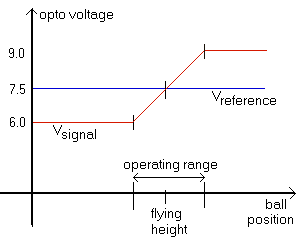### Magnetic Levitation

The sensing resistor is perhaps the single critical component in the levitator. If the value is a bit too high, the coil is always on. If too low the coil stays off.

How do you get just the right value?Read this for help on arriving at the best value for the sensing resistor.

• This graph shows the relation between opto signal (red) and the opto reference (blue). Note that your own voltages will vary from mine.
• Does the difference amp's output (pin 6) go mildly positive, but not nearly to +15 volts? What can happen is that with an object there, the two voltages are practically equal. Here are a few example voltages to see what happens...
• if they're exactly equal then the comparator puts out zero volts, and the coil stays off.
• if the reference is 0.01v LOWER than the signal, then the comparator subtracts the signal from the reference and multiplies by 8, and puts out a negative voltage. This turns off the coil.
• if the reference is 0.01v higher than the signal, then the comparator subtracts the signal from the reference and multiplies by 8, and puts out 0.08 volts. Not enough to turn on the coil much at all. The goal is to have the reference voltage be halfway between the two signal voltages: in your case it should be 12.0 volts.
• Does your levitator work one day, and fail utterly the next? What can happen is one of the sensors shifted. This would move the operating point out of the working range. Do you have the emitter and both detectors firmly secured in place? Either need to shift the sensors slightly to achieve this, or secure them so they can't budge and adjust the 56K resistor to get the right voltage.
• The LED emitter should be adjusted so an equal amount of light falls on both detectors. With an infrared LED you can only guess at this positioning, but if you used a red LED then you can see what to do.
• Measure the voltage on pin 6 of these two op-amps, and fill out this table:
 Signal Detector (volts) Reference Detector (volts) Open ________ ________ Blocked ________ ________

The "open" measurements are taken with nothing under the lifting coil. The "blocked" values are measured with something blocking the light beam, in a position just under the coil.
• If they're already secured, then here's how to adjust the resistor. The goal is to raise the voltage on the sensor by 0.5v. So the resistor needs to be slightly smaller. How much?
- The voltage across the resistor is 15v - 11.5v which is 3.5v.
- We want to make that 0.5v less, or change this to 3.0v.
- Using ratios the new resistance is calculated by R = 56k * (3.0v / 3.5v) = 48K So see if you can come up with a 48K resistor instead of a 56K resistor for the reference detector circuit. That's what will raise the voltage by 0.5v. Please note this will only work if both opto detectors cannot shift around to screw things up again.
• Is this too complicated? Insert a 20K potentiometer in series with the 56K resistor. Twist until it works. You may need to swap it from the signal circuit to the reference circuit, depending on your particular setup. But then again you can't tell if it will ever work without taking the measurements.

If you can get the right value here, then you are well on the way to success! The remaining parts are less critical.

 < Previous Page 32 of 37 Next >